← Back to homepage

# Creating DCGAN with PyTorch

## July 15, 2021 by Chris

Generative Adversarial Networks have been able to produce images that are shockingly realistic (think This Person Does Not Exist). For this reason, I have started focusing on GANs recently. After reading about GAN theory, I wanted to create a GAN myself. For this reason, I started with a relatively simple type of GAN called the Deep Convolutional GAN. In this article, you will...

• Briefly cover what a DCGAN is, to understand what is happening.
• Learn to build a DCGAN with PyTorch.
• See what happens when you train it on the MNIST dataset.

In other words, you're going to build a model that can learn to output what's on the right when beginning with what's on the left:

•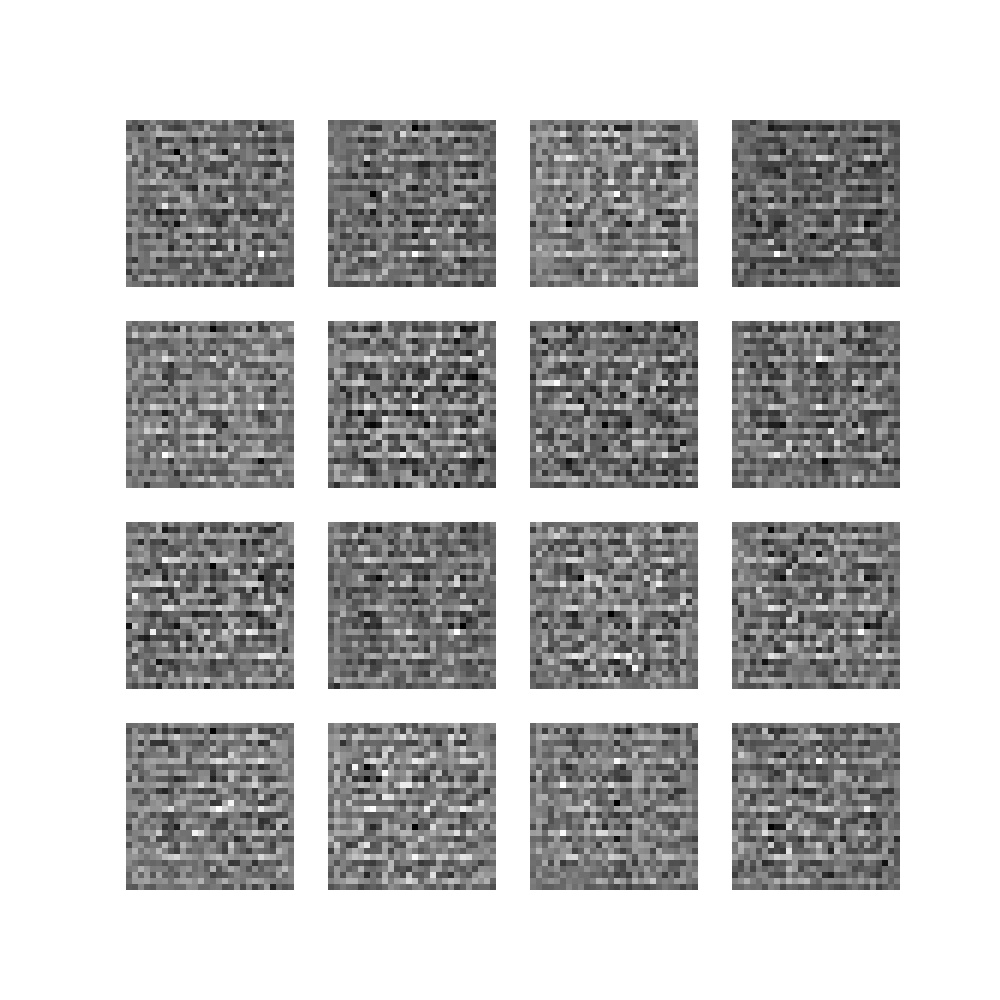•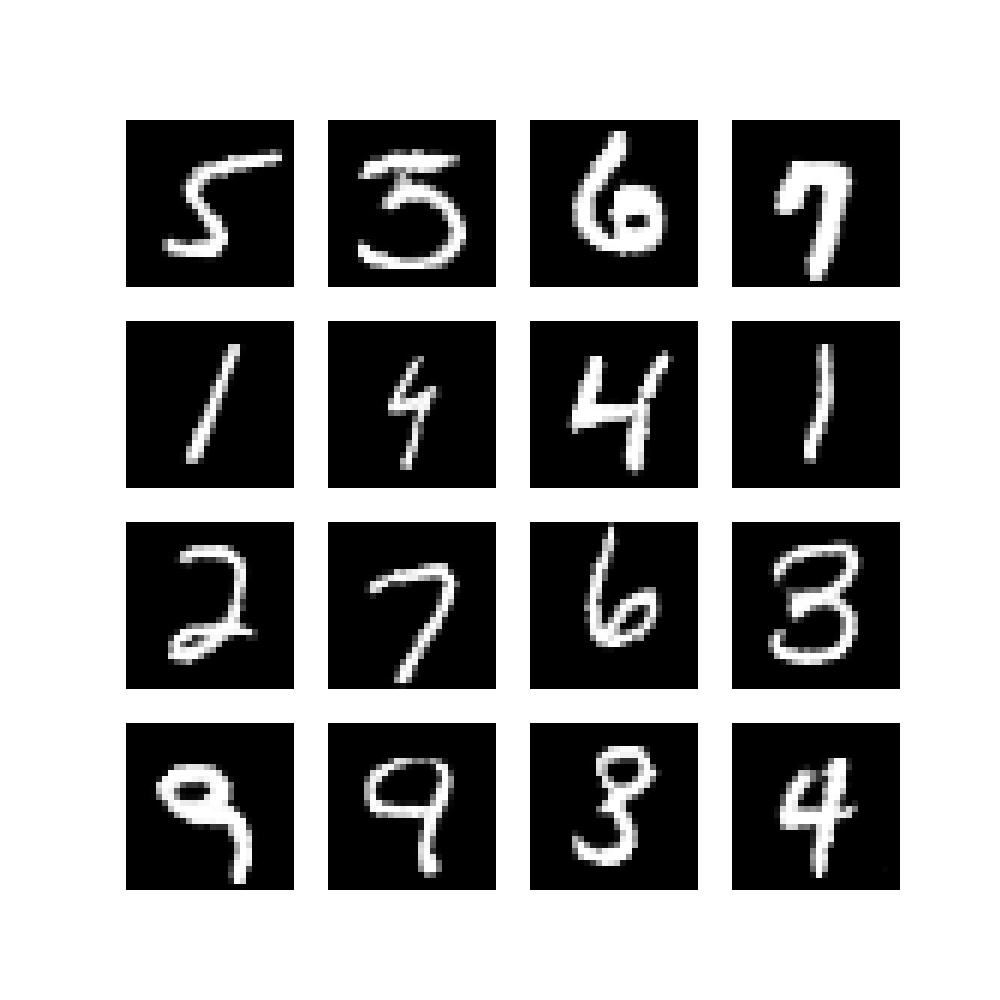Ready? Let's take a look! 😎

## What is a Deep Convolutional GAN (DCGAN)?

A Generative Adversarial Network or GAN for short is a combination of two neural networks and can be used for generative Machine Learning. In other words, and plainer English, it can be used to generate data if it has learned what data it must generate.

As we have seen with This Person Does Not Exist, GANs can be used to generate highly realistic pictures of peoples' faces - because that specific GAN has learned to do so. However, GANs can also be used for more serious purposes, such as composing music for movies and for generative medicine, possibly helping us cure disease.

Now, with respect to the Deep Convolutional GAN that we will create today, we'll briefly cover its components. If you want to understand DCGANs in more detail, refer to this article.

A DCGAN is composed of a Generator and a Discriminator. As you can see in the image below, the Generator takes as input a noise sample, which is taken from a standard normal distribution. It outputs a fake image, which is fed to the Discriminator. The Discriminator itself is trained on real images, and is capable of judging whether the generated image is real or fake. By generating joint loss and subsequent combined optimization, the Discriminator can get better in separating fakes from real, but unknowingly training the Generator in generating better fake images.

Eventually, the noise distribution (also called latent distribution) can be structured in such a way through training, that the Generator generates images that cannot be distinguished anymore, beating the Discriminator. In today's article, we're going to create such a system using PyTorch!

Compared to standard GANs (vanilla GANs / original GANs), DCGANs have a set of additional improvements:

1. A minimum of fully connected layers is used.
2. Any pooling is replaced with learnt downsampling and upsampling.
3. Batch Normalization is applied.
4. ReLU is applied in the Generator.
5. Leaky ReLU is applied in the Discriminator.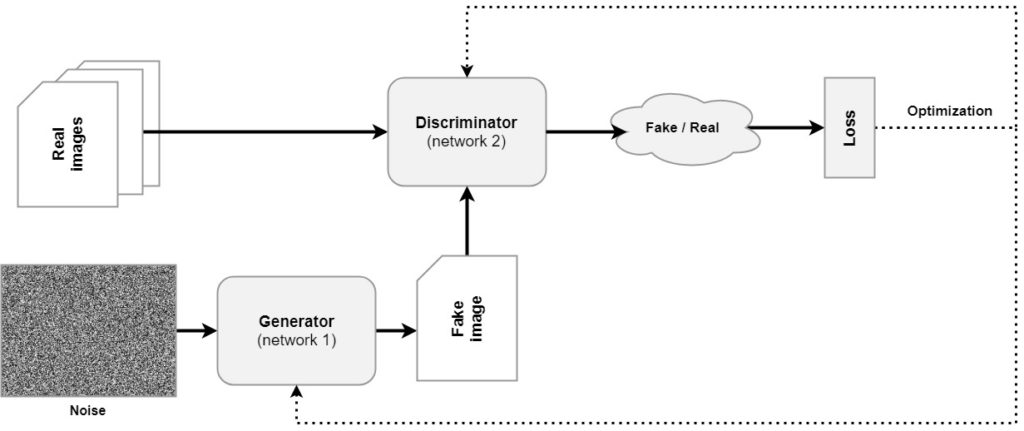## Building a DCGAN with PyTorch

Let's now actually create a Deep Convolutional Gan with PyTorch, including a lot of code examples and step-by-step explanations! :D

### What you'll need to run the code

If you want to run this code, it is important that you have installed the following dependencies:

• PyTorch, including torchvision
• NumPy
• Matplotlib

### Specifying imports and configurable variables

The first step in building a DCGAN is creating a file. Let's call it dcgan.py. We start with specifying the imports:

import os
import torch
from torch import nn
from torchvision.datasets import MNIST
from torchvision import transforms
import numpy as np
import matplotlib.pyplot as plt
import uuid


We import os because we need some Operating System functions. We also import torch and the nn library for building a neural network. As we will train our GAN on the MNIST dataset, we import it, as well as the DataLoader which ensures that the dataset is properly shuffled and batched. The transforms import ensures that we can convert the MNIST images into Tensor format, after which we can normalize them (more on that later).

Finally, we import numpy for some number processing, plt for visualizing the GAN outputs and uuid for generating unique identifiers for each training session - so that we can save the trained models.

This is followed by a variety of configurable variables.

# Configurable variables
NUM_EPOCHS = 50
NOISE_DIMENSION = 50
BATCH_SIZE = 128
TRAIN_ON_GPU = True
UNIQUE_RUN_ID = str(uuid.uuid4())
PRINT_STATS_AFTER_BATCH = 50
OPTIMIZER_LR = 0.0002
OPTIMIZER_BETAS = (0.5, 0.999)

• The number of epochs specifies the number of iterations on the full training set, i.e., the number of epochs.
• The noise dimension can be configured to set the number of dimensions of the noise vector that is input to the Generator.
• The batch size instructs the DataLoader how big batches should be when MNIST samples are loaded.
• If available, we can train on GPU - this can be configured.
• The unique run ID represents a unique identifier that describes this training session, and is used when the models and sample images are saved.
• Print stats after batch tells us how many mini batches should pass in an epoch before intermediary statistics are printed.
• The optimizer LR and optimizer Betas give the Learning Rate and Beta values for the AdamW optimizer used in our GAN.

### Training speedups

PyTorch code can be made to run faster with some simple tweaks. Some must be applied within the model (e.g. in the DataLoader), while others can be applied standalone. Here are some standalone training speedups.

# Speed ups
torch.backends.cudnn.benchmark = True


### The Generator

Now that we have prepared, it's time for the real work! Let's start creating our DCGAN Generator model. Recall that the generator takes a small input sample, generated from a standard normal distribution. It uses Transposed Convolutions (upsampling layers that learn the upsampling process rather than performing interpolation) for constructing the output image in a step-by-step fashion. In our case, the generator produces a 28 x 28 pixel image - hopefully resembling an MNIST digit after a while :)

Below, you'll see the code for the Generator.

• As the Generator is a separate PyTorch model, it must be a class that extends nn.Module.
• In the constructor (__init__), we initialize the superclass, set the number of feature maps output by our model, and create our layers.
• The Generator contains five upsampling blocks. In each, ConvTranspose2d is used for learned upsampling. Starting with the NOISE_DIMENSION (representing the dimensionality of the generated noise), many feature maps (num_feature_maps * 8) are generated, whereas the number of feature maps decreases with downstream layers.
• Note a variety of optimizations:
• Characteristic for DCGAN is the use of Batch Normalization (BatchNorm2d), the use of ReLU in the generator and the use of Tanh after the final upsampling block.
• More generally, bias is set to False in each layer that is followed by a Batch Normalization layer - possibly leading to a model that converges faster. Bias is nullified in a Batch Normalization layer; that's why it makes no sense to use it in the layers directly before BN.
• The forward def simply performs a forward pass.
class Generator(nn.Module):
"""
DCGan Generator
"""
def __init__(self,):
super().__init__()
num_feature_maps = 64
self.layers = nn.Sequential(
# First upsampling block
nn.ConvTranspose2d(NOISE_DIMENSION, num_feature_maps * 8, 4, 1, 0, bias=False),
nn.BatchNorm2d(num_feature_maps * 8),
nn.ReLU(),
# Second upsampling block
nn.ConvTranspose2d(num_feature_maps * 8, num_feature_maps * 4, 4, 2, 1, bias=False),
nn.BatchNorm2d(num_feature_maps * 4),
nn.ReLU(),
# Third upsampling block
nn.ConvTranspose2d(num_feature_maps * 4, num_feature_maps * 2, 4, 2, 1, bias=False),
nn.BatchNorm2d(num_feature_maps * 2),
nn.ReLU(),
# Fourth upsampling block
nn.ConvTranspose2d(num_feature_maps * 2, num_feature_maps, 4, 2, 1, bias=False),
nn.BatchNorm2d(num_feature_maps),
nn.ReLU(),
# Fifth upsampling block: note Tanh
nn.ConvTranspose2d(num_feature_maps, 1, 1, 1, 2, bias=False),
nn.Tanh()
)

def forward(self, x):
"""Forward pass"""
return self.layers(x)


### The Discriminator

Next up: the Discriminator!

Recall that while the Generator generates images, the Discriminator serves as a mechanism of quality control - it can ensure that no fake images pass, and by consequence helps the Generator generate fake images that are difficult to distinguish anymore.

Like the Generator, the Discriminator is also a nn.Module based class with a constructor (__init__) and a forward pass definition (forward). The forward pass def is simple so will not be explained in detail. For the constructor, here's what happens:

• First of all, the number of feature maps is defined. Note that this must be equal to the number of feature maps specified in the Generator.
• It follows the structure of a Convolutional Neural Network. Using a stack of Conv2d layers, feature maps are generated that help detect certain patterns in the input data. The feature maps of the final Conv2d layer are eventually Flattened and passed to a Linear (or fully-connected) layer, after which the Sigmoid activation function ensures that the output is in the range [0, 1].
• Two-dimensional batch normalization (BatchNorm2d) is used to help speed up the training process, as suggested in general and for DCGANs specifically. This is also why, like in the Generator, the bias values for the preceding layers are set to False.
• Leaky ReLU with an alpha=0.2 is used instead of regular ReLU.
class Discriminator(nn.Module):
"""
DCGan Discriminator
"""
def __init__(self):
super().__init__()
num_feature_maps = 64
self.layers = nn.Sequential(
nn.Conv2d(1, num_feature_maps, 4, 2, 1, bias=False),
nn.BatchNorm2d(num_feature_maps * 1),
nn.LeakyReLU(0.2),
nn.Conv2d(num_feature_maps, num_feature_maps * 2, 4, 2, 1, bias=False),
nn.BatchNorm2d(num_feature_maps * 2),
nn.LeakyReLU(0.2),
nn.Conv2d(num_feature_maps * 2, num_feature_maps * 4, 4, 2, 1, bias=False),
nn.BatchNorm2d(num_feature_maps * 4),
nn.LeakyReLU(0.2),
nn.Conv2d(num_feature_maps * 4, 1, 4, 2, 1, bias=False),
nn.Flatten(),
nn.Linear(1, 1),
nn.Sigmoid()
)

def forward(self, x):
"""Forward pass"""
return self.layers(x)


### The DCGAN: a set of definitions

Now that we have built the Generator and the Discriminator, it's actually time to construct functionality for the DCGAN! :)

In Python, it's good practice to split as many individual functionalities in separate definitions. This avoids that you'll end up with one large block of code, and ensures that you can re-use certain functions if they must be used in different places.

Now, because a GAN is quite complex in terms of functionality, we're going to write a lot of definitions that eventually will be merged together:

• Getting the PyTorch device: recall that you used TRAIN_ON_GPU for specifying whether you want to train the GAN on your GPU or your CPU. Getting the PyTorch device makes sure that all other functionalities can use that device, should it be available.
• Making run directory & generating images: the GAN will be constructed in such a way, that each time when you run it, a folder is created with a UNIQUE_RUN_ID. Here, saved models and images generated during the training process will be stored. We'll also construct the def for generating the images there. House keeping, in other words.
• Functionality for saving models & printing progress: even more house keeping. Models will be saved every once in a while, so that intermediate versions of your GAN can also be used. Here, we'll create a def for that, as well as a def for printing progress during the training process.
• Preparing the dataset: now that we have written defs for house keeping, we can continue with the real work. Next up is a def for preparing the MNIST dataset.
• Weight initializer function: DCGAN weights must be initialized in a specific way. With this def, you'll ensure that this is done properly.
• Initializing models, loss and optimizers: here, we will create three defs for initializing the models (i.e. Generator and Discriminator), loss function (BCELoss) and optimizers (we will use AdamW, which is Adam with weight decay).
• Generating noise: recall that Generators take noise from a latent distribution and convert it into an output image. We create a def that can generate noise.
• Efficiently zero-ing gradients: in PyTorch, gradients must be zeroed before a new training step can occur. While PyTorch has zero_grad() for this, it can be done more efficiently. We create a custom def for this purpose.
• Forward and backward passes: the real work! Here, we feed a batch of data through a model, perform backpropagation and return the loss. The def will be set up in a generic way so that it can be used for both Generator and Discriminator.
• Combining passes into a training step: each batch of data will be forward and backward propagated. This def ensures that the previous def will be called for the batch of data currently being enumerated.
• Combining training steps into epochs: recall that one epoch is a forward and backward pass over all the training data. In other words, one epoch combines all training steps for the training data. In this def, we ensure that this happens.
• Combining epochs into a DCGAN: the final def that combines everything. It calls previous defs for preparation and eventually starts the training process by iterating according to the number of epochs configured before.

Let's now take a look at all the definitions and provide example code.

#### Getting the PyTorch device

In this def, we'll construct the PyTorch device depending on configuration and availability. We check whether both TRAIN_ON_GPU and torch.cuda.is_available() resolve to True to ensure that the cuda:0 device can be loaded. If not, we use our CPU.

Note that this def is configured to run the GAN on one GPU only. You'll have to manually add a multi-GPU training strategy if necessary.

def get_device():
""" Retrieve device based on settings and availability. """


#### Making run directory & generating images

The contents of make_directory_for_run(), the definition that makes a directory for the training run, are quite straight-forward. It checks whether a folder called runs exists in the current path, and creates it if it isn't available. Then, in ./runs, a folder for the UNIQUE_RUN_ID is created.

def make_directory_for_run():
""" Make a directory for this training run. """
print(f'Preparing training run {UNIQUE_RUN_ID}')
if not os.path.exists('./runs'):
os.mkdir('./runs')
os.mkdir(f'./runs/{UNIQUE_RUN_ID}')


In generate_image, an image with sub plots containing generated examples will be created. As you can see, noise is generated, fed to the generator, and is then added to a Matplotlib plot. It is saved to a folder images relative to /.runs/{UNIQUE_RUN_ID} which itself is created if it doesn't exist yet.

def generate_image(generator, epoch = 0, batch = 0, device=get_device()):
""" Generate subplots with generated examples. """
images = []
noise = generate_noise(BATCH_SIZE, device=device)
generator.eval()
images = generator(noise)
plt.figure(figsize=(10, 10))
for i in range(16):
# Get image
image = images[i]
# Convert image back onto CPU and reshape
image = image.cpu().detach().numpy()
image = np.reshape(image, (28, 28))
# Plot
plt.subplot(4, 4, i+1)
plt.imshow(image, cmap='gray')
plt.axis('off')
if not os.path.exists(f'./runs/{UNIQUE_RUN_ID}/images'):
os.mkdir(f'./runs/{UNIQUE_RUN_ID}/images')
plt.savefig(f'./runs/{UNIQUE_RUN_ID}/../../../../static/images/epoch{epoch}_batch{batch}.jpg')


#### Functionality for saving models & printing progress

Saving the models is also really straight-forward. Once again, they are saved relative to ./runs/{UNIQUE_RUN_ID}, and both the generator and discriminator are saved. As they are saved after every epoch ends, the epoch is passed as well and included in the *.pth file.

def save_models(generator, discriminator, epoch):
""" Save models at specific point in time. """
torch.save(generator.state_dict(), f'./runs/{UNIQUE_RUN_ID}/generator_{epoch}.pth')
torch.save(discriminator.state_dict(), f'./runs/{UNIQUE_RUN_ID}/discriminator_{epoch}.pth')


Printing training progress during the training steps is done with a specific def called print_training_progress. It simply prints the batch number, generator loss and discriminator loss in a standardized way.

def print_training_progress(batch, generator_loss, discriminator_loss):
""" Print training progress. """
print('Losses after mini-batch %5d: generator %e, discriminator %e' %
(batch, generator_loss, discriminator_loss))


#### Preparing the dataset

Recall that all previous definitions were preparatory in terms of house keeping, but that you will now create a definition for preparing the dataset. It is as follows:

def prepare_dataset():
""" Prepare dataset through DataLoader """
# Prepare MNIST dataset
transforms.ToTensor(),
transforms.Normalize((0.5,), (0.5,))
]))
# Batch and shuffle data with DataLoader


Here, you can see that the MNIST dataset is loaded from the current working directory (os.getcwd()). It is downloaded if necessary and the training data is used. In addition, a composition of various transforms is used. First, we convert the MNIST images (which are PIL-based images) into Tensor format, so that PyTorch can use them efficiently. Subsequently, the images are Normalized into the range [-1, 1].

That the dataset is now available does not mean that it can already be used. We must apply a DataLoader to use the data efficiently; in other words, in batches (hence BATCH_SIZE) and in a shuffled fashion. The number of workers is set to 4 and memory is pinned due to the PyTorch efficiencies that we discussed earlier.

Finally, the dataloader is returned.

#### Weight initializer function

Recall from the Radford et al. (2015) paper that weights must be initialized in a specific way:

All weights were initialized from a zero-centered Normal distribution with standard deviation 0.02.

Next, we therefore write a definition that ensures this and which can be used later:

def weights_init(m):
""" Normal weight initialization as suggested for DCGANs """
classname = m.__class__.__name__
if classname.find('Conv') != -1:
nn.init.normal_(m.weight.data, 0.0, 0.02)
elif classname.find('BatchNorm') != -1:
nn.init.normal_(m.weight.data, 1.0, 0.02)
nn.init.constant_(m.bias.data, 0)


#### Initializing models, loss and optimizers

Now, we create three definitions:

• In initialize_models(), we initialize the Generator and the Discriminator. Here, the weights_init def is also applied, and the models are moved to the device that was configured. Both the Generator and Discriminator are then returned.
• Using initialize_loss, an instance of Binary cross-entropy loss is returned. BCELoss is used to compare an output between 0 and 1 with a corresponding target variable, which is either 0 or 1.
• With initialize_optimizers, we init the optimizers for both the Generator and the Discriminator. Recall that each is an individual neural network and hence requires a separate optimizer. We use AdamW, which is Adam with weight decay - it is expected to make training faster. The learning rates and optimizer betas are configured in line with configuration options specified above.
def initialize_models(device = get_device()):
""" Initialize Generator and Discriminator models """
generator = Generator()
discriminator = Discriminator()
# Perform proper weight initialization
generator.apply(weights_init)
discriminator.apply(weights_init)
# Move models to specific device
generator.to(device)
discriminator.to(device)
# Return models
return generator, discriminator

def initialize_loss():
""" Initialize loss function. """
return nn.BCELoss()

def initialize_optimizers(generator, discriminator):
""" Initialize optimizers for Generator and Discriminator. """
return generator_optimizer, discriminator_optimizer


#### Generating noise

The definition for generating noise is also really straight-forward. Using torch.rand, noise for a specific amount of images with a specific dimension is generated into a specific device.

def generate_noise(number_of_images = 1, noise_dimension = NOISE_DIMENSION, device=None):
""" Generate noise for number_of_images images, with a specific noise_dimension """


In PyTorch, gradients must be zeroed during every training step because otherwise history can interfire with the current training step. PyTorch itself provides zero_grad() for this purpose, but it sets gradients to 0.0 - which is numeric rather than None. It was found that setting the gradients to None can make training faster. Hence, we create a definition for thus purpose, which can be used with any model and can be re-used multiple times later in this article.

def efficient_zero_grad(model):
"""
Source: https://betterprogramming.pub/how-to-make-your-pytorch-code-run-faster-93079f3c1f7b
"""
for param in model.parameters():


#### Forward and backward passes

Recall that training a neural network involves a forward pass, where data is passed through the network returning predictions, and a backward pass, where the error is backpropagated through the network. Once this is done, the network can be optimized. In this definition, we ensure that for any model a batch of data can be fed forward through the model. Subsequently, using a loss_function, loss is computed and subsequently backpropagated through the network. The numeric value for loss is returned so that it can be printed with the print def created above.

def forward_and_backward(model, data, loss_function, targets):
"""
Perform forward and backward pass in a generic way. Returns loss value.
"""
outputs = model(data)
error = loss_function(outputs, targets)
error.backward()
return error.item()


#### Combining the passes into a training step

So far, we have created everything that is necessary for constructing functionality for a single training step. Recall that training the GAN involves iterating for a specific amount of epochs, and that each epoch is composed of a number of training steps.

Here, you will create the def for the training steps. As you can see, the generator, discriminator, a batch of real_data, as well as loss functions and optimizers can be passed. A specific device can be passed as well, or the configured device will be used.

A training step consists of four phrases:

1. Preparation. Here, the real and fake labels are set, the real images are loaded onto the device, and a Tensor with the real label is set so that we can train the discriminator with real images.
2. Training the discriminator. First, the gradients are zeroed, after which a forward and backward pass is performed with the discriminator and real images. Directly afterwards, a forward and backward pass is performed on an equal amount of fake images, for which noise is generated. After these passes, the discriminator is optimized.
3. Training the generator. This involves a forward pass on the generated images for the updated discriminator, after which the generator is optimized with resulting loss. Here you can see the interplay between discriminator and generator: the discriminator is first updated based on images generated by the generator (using its current state), after which the generator is trained based on the updated discriminator. In other words, they play the minimax game which is characteristic for a GAN.
4. Computing the results. Finally, some results are computed, and loss values for the discriminator and generator are returned.
def perform_train_step(generator, discriminator, real_data, \
loss_function, generator_optimizer, discriminator_optimizer, device = get_device()):
""" Perform a single training step. """

# 1. PREPARATION
# Set real and fake labels.
real_label, fake_label = 1.0, 0.0
# Get images on CPU or GPU as configured and available
# Also set 'actual batch size', whih can be smaller than BATCH_SIZE
# in some cases.
real_images = real_data.to(device)
actual_batch_size = real_images.size(0)
label = torch.full((actual_batch_size,1), real_label, device=device)

# 2. TRAINING THE DISCRIMINATOR
# Zero the gradients for discriminator
# Forward + backward on real iamges
error_real_images = forward_and_backward(discriminator, real_images, \
loss_function, label)
# Forward + backward on generated images
noise = generate_noise(actual_batch_size, device=device)
generated_images = generator(noise)
label.fill_(fake_label)
error_generated_images =forward_and_backward(discriminator, \
generated_images.detach(), loss_function, label)
# Optim for discriminator
discriminator_optimizer.step()

# 3. TRAINING THE GENERATOR
# Forward + backward + optim for generator, including zero grad
label.fill_(real_label)
error_generator = forward_and_backward(discriminator, generated_images, loss_function, label)
generator_optimizer.step()

# 4. COMPUTING RESULTS
# Compute loss values in floats for discriminator, which is joint loss.
error_discriminator = error_real_images + error_generated_images
# Return generator and discriminator loss so that it can be printed.
return error_generator, error_discriminator


#### Combining training steps into epochs

Recall that an epoch consists of multiple training steps. With the perform_epoch def, we iterate over the data provided by the dataloader. For each batch of real_data, we perform the training step by calling perform_train_step that we just created above. After each training step is completed, we check if a certain amount of steps has been completed after which we print the training progress and generate intermediate images.

On epoch completion, the generator and discriminator are saved and CUDA memory is cleared as far as possible, speeding up the training process.

def perform_epoch(dataloader, generator, discriminator, loss_function, \
generator_optimizer, discriminator_optimizer, epoch):
""" Perform a single epoch. """
for batch_no, real_data in enumerate(dataloader, 0):
# Perform training step
generator_loss_val, discriminator_loss_val = perform_train_step(generator, \
discriminator, real_data, loss_function, \
generator_optimizer, discriminator_optimizer)
# Print statistics and generate image after every n-th batch
if batch_no % PRINT_STATS_AFTER_BATCH == 0:
print_training_progress(batch_no, generator_loss_val, discriminator_loss_val)
generate_image(generator, epoch, batch_no)
# Save models on epoch completion.
save_models(generator, discriminator, epoch)
# Clear memory after every epoch
torch.cuda.empty_cache()


#### Combining epochs into a DCGAN

Now that you have completed the preparatory definitions, the training step and the epochs, it's time to actually combine everything into a definition that allows us to train the GAN.

In the code below, you can see that a directory for the training run is created, the random seet is configured, the dataset is prepared, that models, loss and optimizers are initialized, and that finally the model is trained per perform_epoch (and hence per the training steps).

def train_dcgan():
""" Train the DCGAN. """
# Make directory for unique run
make_directory_for_run()
# Set fixed random number seed
torch.manual_seed(42)
# Get prepared dataset
# Initialize models
generator, discriminator = initialize_models()
# Initialize loss and optimizers
loss_function = initialize_loss()
generator_optimizer, discriminator_optimizer = initialize_optimizers(generator, discriminator)
# Train the model
for epoch in range(NUM_EPOCHS):
print(f'Starting epoch {epoch}...')
generator_optimizer, discriminator_optimizer, epoch)
# Finished :-)
print(f'Finished unique run {UNIQUE_RUN_ID}')


### Initializing and starting GAN training

There is only one thing left now, and that is to instruct Python to call the train_dcgan() definition when you run the script:

if __name__ == '__main__':
train_dcgan()


### Full DCGAN code example

Of course, it is also possible to copy and use the DCGAN code altogether. If that's what you want, here you go:

import os
import torch
from torch import nn
from torchvision.datasets import MNIST
from torchvision import transforms
import numpy as np
import matplotlib.pyplot as plt
import uuid

# Configurable variables
NUM_EPOCHS = 50
NOISE_DIMENSION = 50
BATCH_SIZE = 128
TRAIN_ON_GPU = True
UNIQUE_RUN_ID = str(uuid.uuid4())
PRINT_STATS_AFTER_BATCH = 50
OPTIMIZER_LR = 0.0002
OPTIMIZER_BETAS = (0.5, 0.999)

# Speed ups
torch.backends.cudnn.benchmark = True

class Generator(nn.Module):
"""
DCGan Generator
"""
def __init__(self,):
super().__init__()
num_feature_maps = 64
self.layers = nn.Sequential(
# First upsampling block
nn.ConvTranspose2d(NOISE_DIMENSION, num_feature_maps * 8, 4, 1, 0, bias=False),
nn.BatchNorm2d(num_feature_maps * 8),
nn.ReLU(),
# Second upsampling block
nn.ConvTranspose2d(num_feature_maps * 8, num_feature_maps * 4, 4, 2, 1, bias=False),
nn.BatchNorm2d(num_feature_maps * 4),
nn.ReLU(),
# Third upsampling block
nn.ConvTranspose2d(num_feature_maps * 4, num_feature_maps * 2, 4, 2, 1, bias=False),
nn.BatchNorm2d(num_feature_maps * 2),
nn.ReLU(),
# Fourth upsampling block
nn.ConvTranspose2d(num_feature_maps * 2, num_feature_maps, 4, 2, 1, bias=False),
nn.BatchNorm2d(num_feature_maps),
nn.ReLU(),
# Fifth upsampling block: note Tanh
nn.ConvTranspose2d(num_feature_maps, 1, 1, 1, 2, bias=False),
nn.Tanh()
)

def forward(self, x):
"""Forward pass"""
return self.layers(x)

class Discriminator(nn.Module):
"""
DCGan Discriminator
"""
def __init__(self):
super().__init__()
num_feature_maps = 64
self.layers = nn.Sequential(
nn.Conv2d(1, num_feature_maps, 4, 2, 1, bias=False),
nn.BatchNorm2d(num_feature_maps * 1),
nn.LeakyReLU(0.2),
nn.Conv2d(num_feature_maps, num_feature_maps * 2, 4, 2, 1, bias=False),
nn.BatchNorm2d(num_feature_maps * 2),
nn.LeakyReLU(0.2),
nn.Conv2d(num_feature_maps * 2, num_feature_maps * 4, 4, 2, 1, bias=False),
nn.BatchNorm2d(num_feature_maps * 4),
nn.LeakyReLU(0.2),
nn.Conv2d(num_feature_maps * 4, 1, 4, 2, 1, bias=False),
nn.Flatten(),
nn.Linear(1, 1),
nn.Sigmoid()
)

def forward(self, x):
"""Forward pass"""
return self.layers(x)

def get_device():
""" Retrieve device based on settings and availability. """

def make_directory_for_run():
""" Make a directory for this training run. """
print(f'Preparing training run {UNIQUE_RUN_ID}')
if not os.path.exists('./runs'):
os.mkdir('./runs')
os.mkdir(f'./runs/{UNIQUE_RUN_ID}')

def generate_image(generator, epoch = 0, batch = 0, device=get_device()):
""" Generate subplots with generated examples. """
images = []
noise = generate_noise(BATCH_SIZE, device=device)
generator.eval()
images = generator(noise)
plt.figure(figsize=(10, 10))
for i in range(16):
# Get image
image = images[i]
# Convert image back onto CPU and reshape
image = image.cpu().detach().numpy()
image = np.reshape(image, (28, 28))
# Plot
plt.subplot(4, 4, i+1)
plt.imshow(image, cmap='gray')
plt.axis('off')
if not os.path.exists(f'./runs/{UNIQUE_RUN_ID}/images'):
os.mkdir(f'./runs/{UNIQUE_RUN_ID}/images')
plt.savefig(f'./runs/{UNIQUE_RUN_ID}/../../../../static/images/epoch{epoch}_batch{batch}.jpg')

def save_models(generator, discriminator, epoch):
""" Save models at specific point in time. """
torch.save(generator.state_dict(), f'./runs/{UNIQUE_RUN_ID}/generator_{epoch}.pth')
torch.save(discriminator.state_dict(), f'./runs/{UNIQUE_RUN_ID}/discriminator_{epoch}.pth')

def print_training_progress(batch, generator_loss, discriminator_loss):
""" Print training progress. """
print('Losses after mini-batch %5d: generator %e, discriminator %e' %
(batch, generator_loss, discriminator_loss))

def prepare_dataset():
""" Prepare dataset through DataLoader """
# Prepare MNIST dataset
transforms.ToTensor(),
transforms.Normalize((0.5,), (0.5,))
]))
# Batch and shuffle data with DataLoader

def weights_init(m):
""" Normal weight initialization as suggested for DCGANs """
classname = m.__class__.__name__
if classname.find('Conv') != -1:
nn.init.normal_(m.weight.data, 0.0, 0.02)
elif classname.find('BatchNorm') != -1:
nn.init.normal_(m.weight.data, 1.0, 0.02)
nn.init.constant_(m.bias.data, 0)

def initialize_models(device = get_device()):
""" Initialize Generator and Discriminator models """
generator = Generator()
discriminator = Discriminator()
# Perform proper weight initialization
generator.apply(weights_init)
discriminator.apply(weights_init)
# Move models to specific device
generator.to(device)
discriminator.to(device)
# Return models
return generator, discriminator

def initialize_loss():
""" Initialize loss function. """
return nn.BCELoss()

def initialize_optimizers(generator, discriminator):
""" Initialize optimizers for Generator and Discriminator. """
return generator_optimizer, discriminator_optimizer

def generate_noise(number_of_images = 1, noise_dimension = NOISE_DIMENSION, device=None):
""" Generate noise for number_of_images images, with a specific noise_dimension """

"""
Source: https://betterprogramming.pub/how-to-make-your-pytorch-code-run-faster-93079f3c1f7b
"""
for param in model.parameters():

def forward_and_backward(model, data, loss_function, targets):
"""
Perform forward and backward pass in a generic way. Returns loss value.
"""
outputs = model(data)
error = loss_function(outputs, targets)
error.backward()
return error.item()

def perform_train_step(generator, discriminator, real_data, \
loss_function, generator_optimizer, discriminator_optimizer, device = get_device()):
""" Perform a single training step. """

# 1. PREPARATION
# Set real and fake labels.
real_label, fake_label = 1.0, 0.0
# Get images on CPU or GPU as configured and available
# Also set 'actual batch size', whih can be smaller than BATCH_SIZE
# in some cases.
real_images = real_data.to(device)
actual_batch_size = real_images.size(0)
label = torch.full((actual_batch_size,1), real_label, device=device)

# 2. TRAINING THE DISCRIMINATOR
# Zero the gradients for discriminator
# Forward + backward on real iamges
error_real_images = forward_and_backward(discriminator, real_images, \
loss_function, label)
# Forward + backward on generated images
noise = generate_noise(actual_batch_size, device=device)
generated_images = generator(noise)
label.fill_(fake_label)
error_generated_images =forward_and_backward(discriminator, \
generated_images.detach(), loss_function, label)
# Optim for discriminator
discriminator_optimizer.step()

# 3. TRAINING THE GENERATOR
# Forward + backward + optim for generator, including zero grad
label.fill_(real_label)
error_generator = forward_and_backward(discriminator, generated_images, loss_function, label)
generator_optimizer.step()

# 4. COMPUTING RESULTS
# Compute loss values in floats for discriminator, which is joint loss.
error_discriminator = error_real_images + error_generated_images
# Return generator and discriminator loss so that it can be printed.
return error_generator, error_discriminator

def perform_epoch(dataloader, generator, discriminator, loss_function, \
generator_optimizer, discriminator_optimizer, epoch):
""" Perform a single epoch. """
for batch_no, real_data in enumerate(dataloader, 0):
# Perform training step
generator_loss_val, discriminator_loss_val = perform_train_step(generator, \
discriminator, real_data, loss_function, \
generator_optimizer, discriminator_optimizer)
# Print statistics and generate image after every n-th batch
if batch_no % PRINT_STATS_AFTER_BATCH == 0:
print_training_progress(batch_no, generator_loss_val, discriminator_loss_val)
generate_image(generator, epoch, batch_no)
# Save models on epoch completion.
save_models(generator, discriminator, epoch)
# Clear memory after every epoch
torch.cuda.empty_cache()

def train_dcgan():
""" Train the DCGAN. """
# Make directory for unique run
make_directory_for_run()
# Set fixed random number seed
torch.manual_seed(42)
# Get prepared dataset
# Initialize models
generator, discriminator = initialize_models()
# Initialize loss and optimizers
loss_function = initialize_loss()
generator_optimizer, discriminator_optimizer = initialize_optimizers(generator, discriminator)
# Train the model
for epoch in range(NUM_EPOCHS):
print(f'Starting epoch {epoch}...')
generator_optimizer, discriminator_optimizer, epoch)
# Finished :-)
print(f'Finished unique run {UNIQUE_RUN_ID}')

if __name__ == '__main__':
train_dcgan()


## Results

Time to start the training process! Ensure that the dependencies listed above are installed in your environment, open up a terminal, and run python dcgan.py. You should see that the process starts when these messages start showing up on your screen:

Preparing training run bbc1b297-fd9d-4a01-abc6-c4d03f18d54f
Starting epoch 0...
Losses after mini-batch     0: generator 1.337156e+00, discriminator 1.734429e+00
Losses after mini-batch    50: generator 3.972991e+00, discriminator 1.365001e-01
Losses after mini-batch   100: generator 4.795033e+00, discriminator 3.830627e-02
Losses after mini-batch   150: generator 5.441184e+00, discriminator 1.489213e-02
Losses after mini-batch   200: generator 5.729664e+00, discriminator 1.159845e-02
Losses after mini-batch   250: generator 5.579849e+00, discriminator 1.056747e-02
Losses after mini-batch   300: generator 5.983423e+00, discriminator 5.716243e-03
Losses after mini-batch   350: generator 6.004053e+00, discriminator 6.531999e-03
Losses after mini-batch   400: generator 2.578202e+00, discriminator 3.643379e-01
Losses after mini-batch   450: generator 4.946642e+00, discriminator 3.067930e-01
Starting epoch 1...


Don't worry if you'll see the model produce nonsense for the first series of batches. Only after 400 batches in the first epoch the model started to produce something that something good was happening :)

•Untrained model

•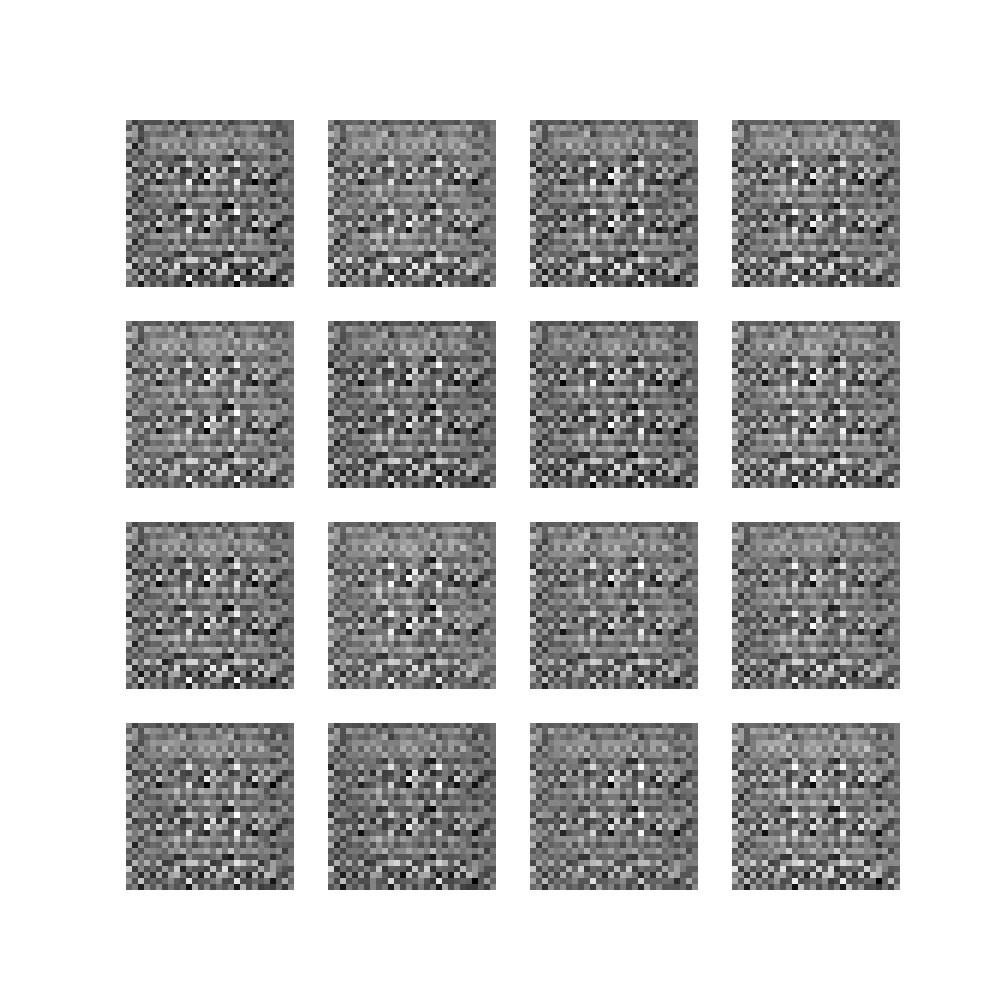Epoch 0, batch 100

•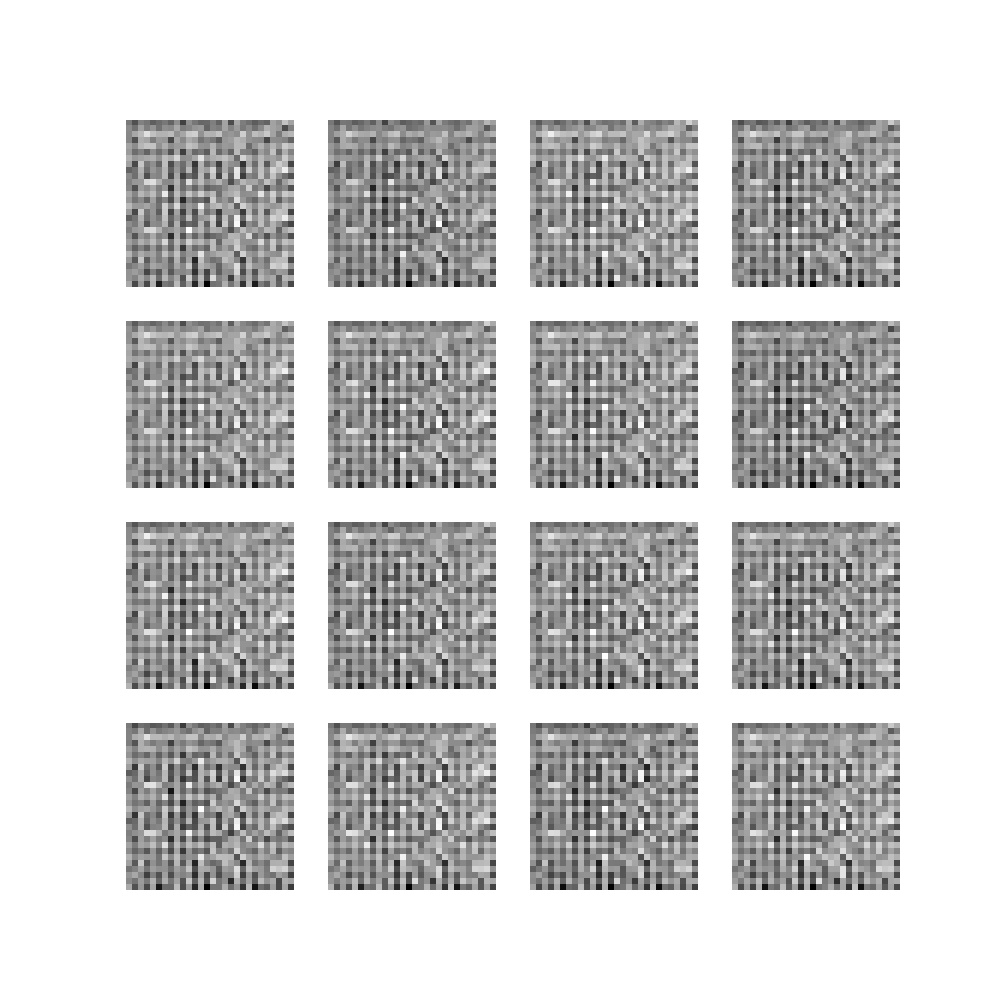Epoch 0, batch 200

•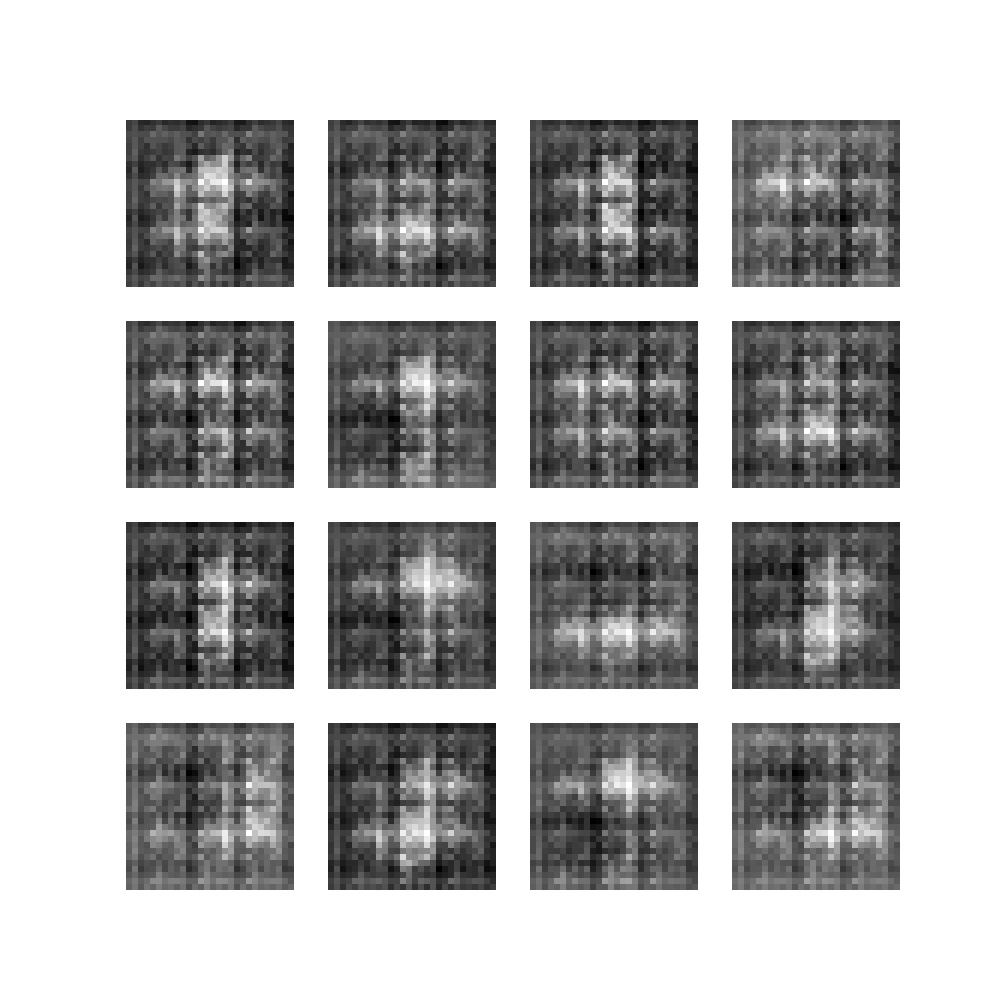Epoch 0, batch 300

•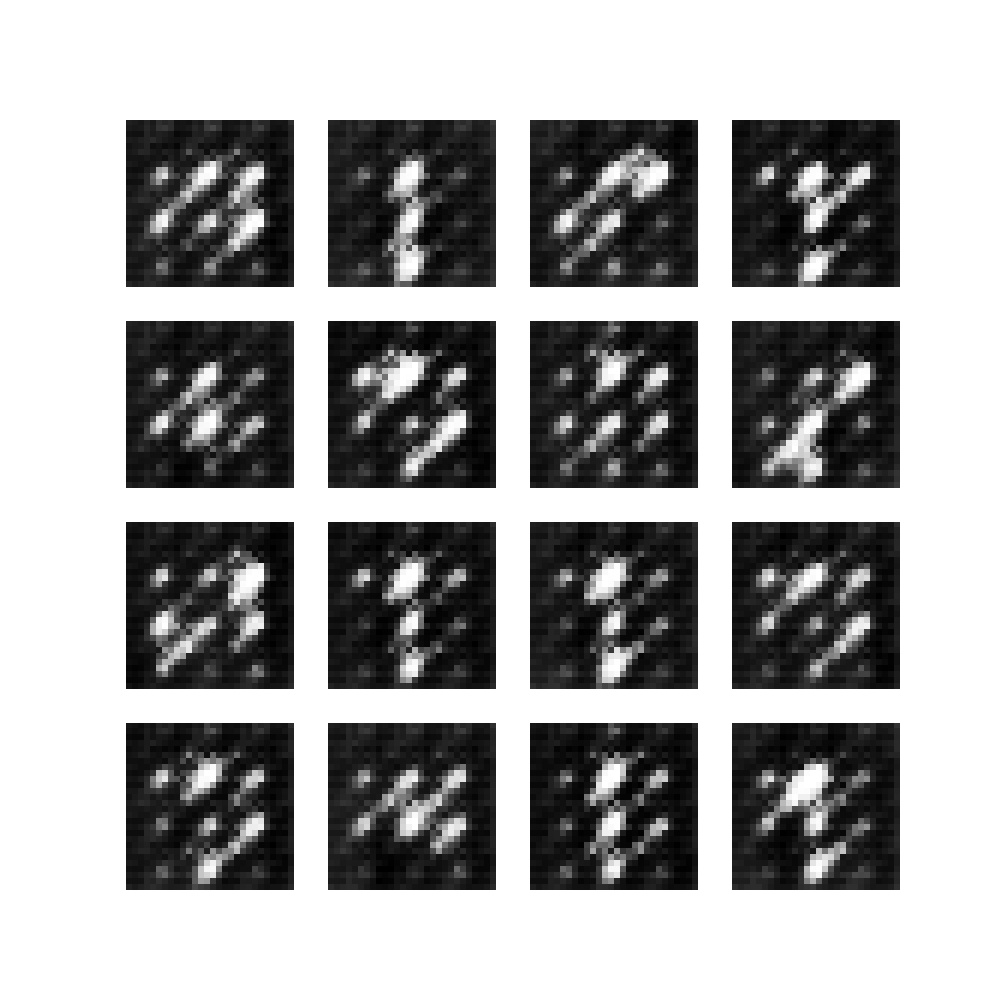Epoch 0, batch 400

•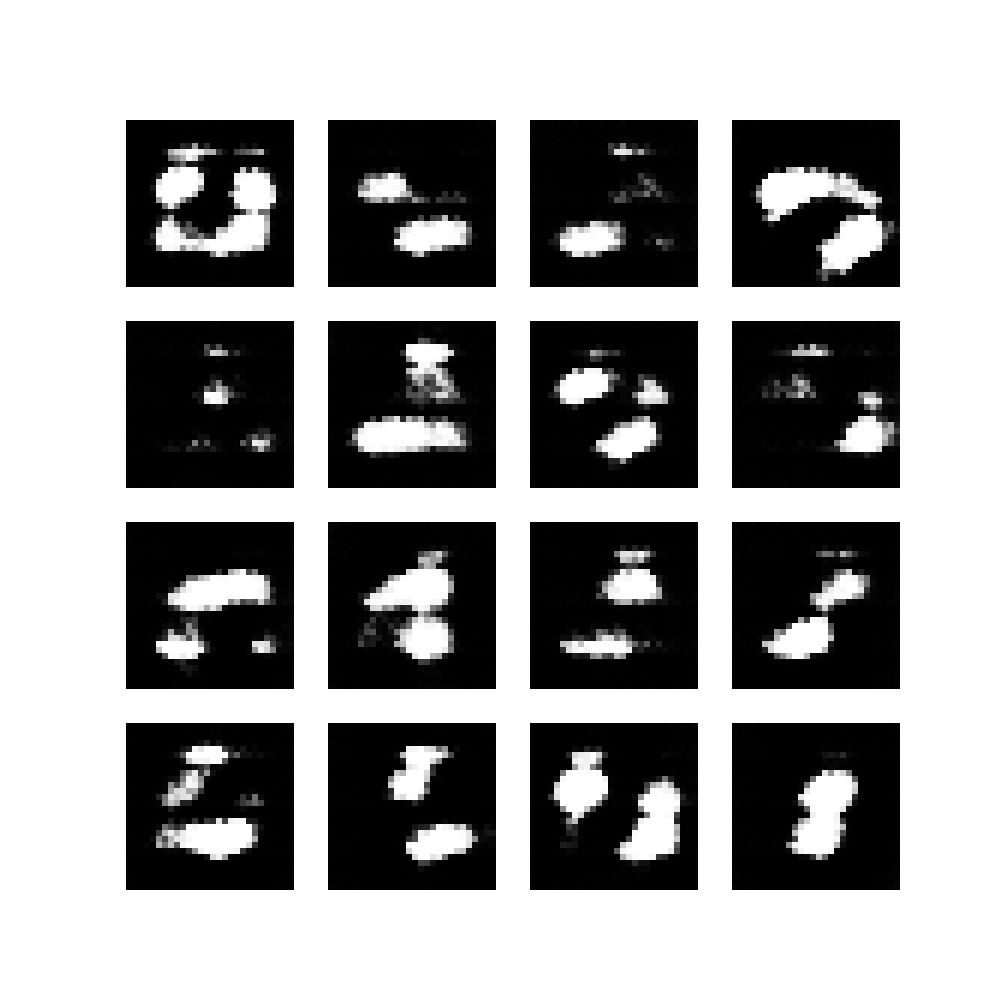Epoch 1, batch 0

•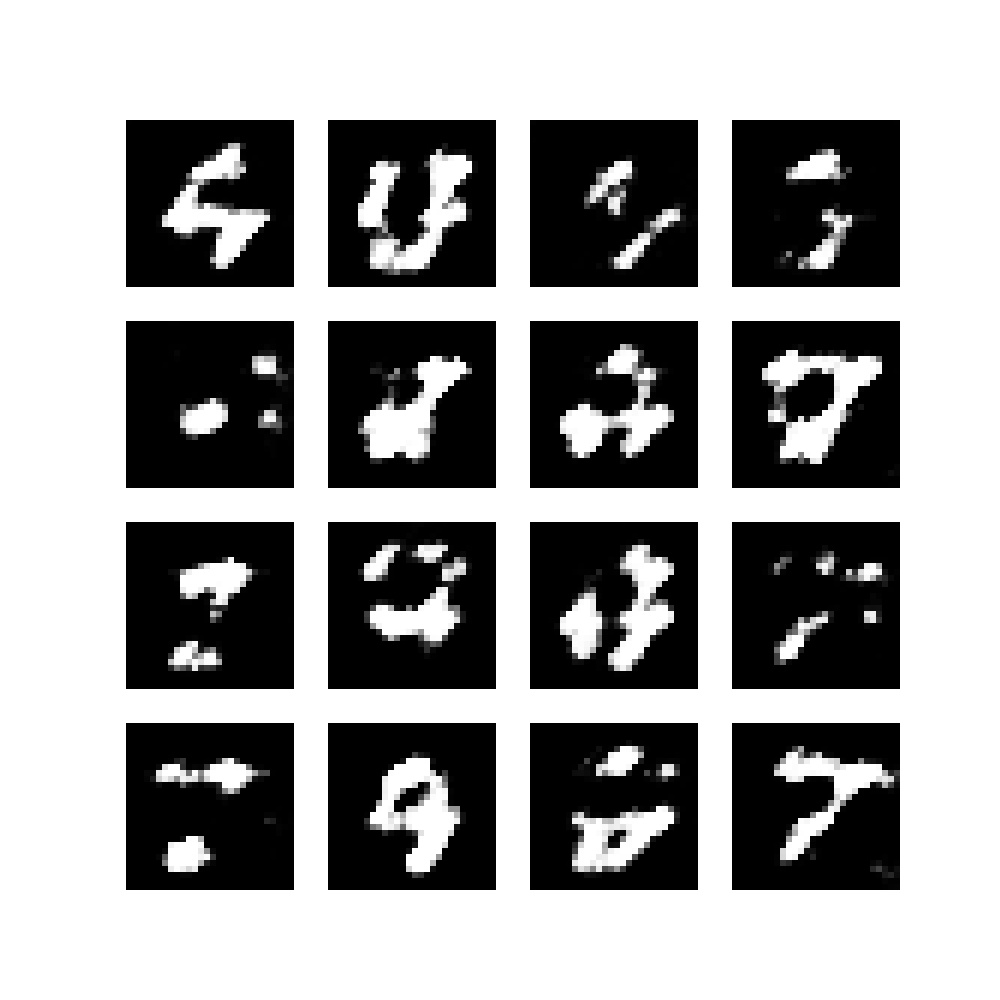Epoch 1, batch 100

•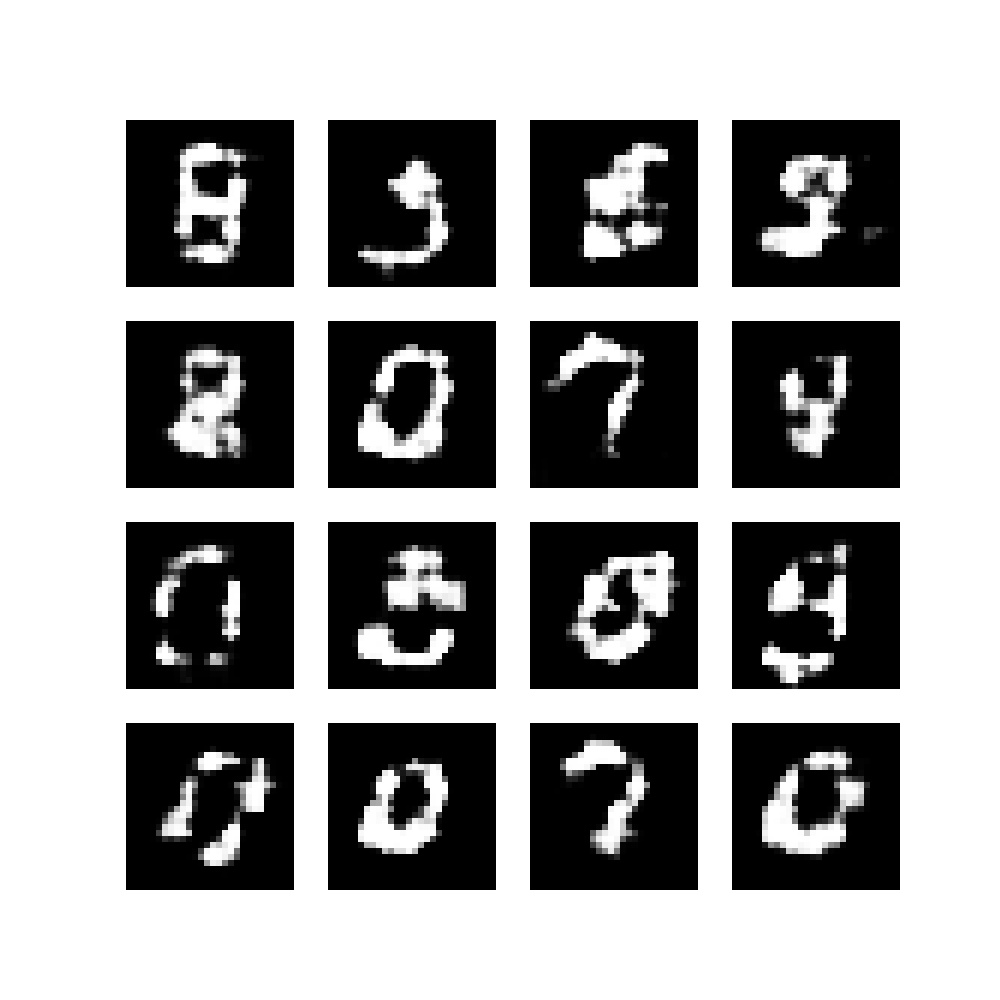Epoch 1, batch 200

•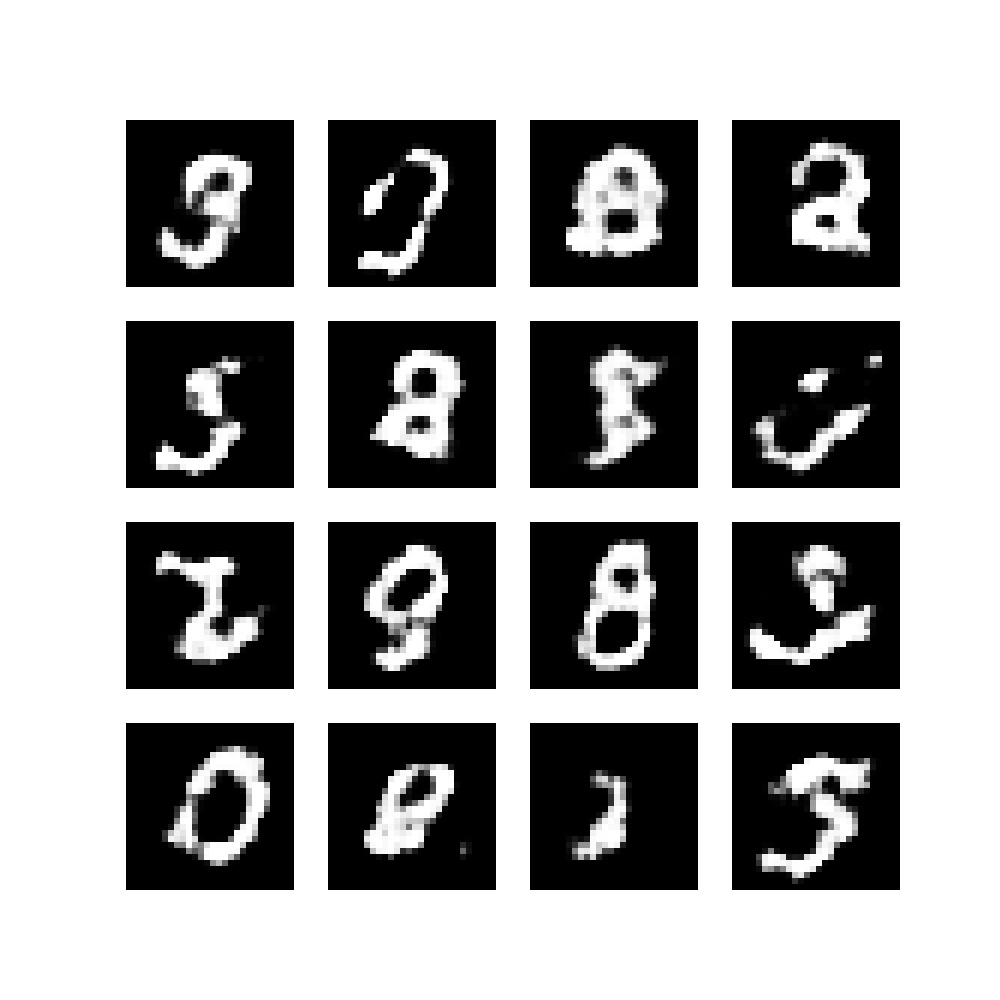Epoch 1, batch 300

After epoch 22, the numbers were already becoming realistic:

•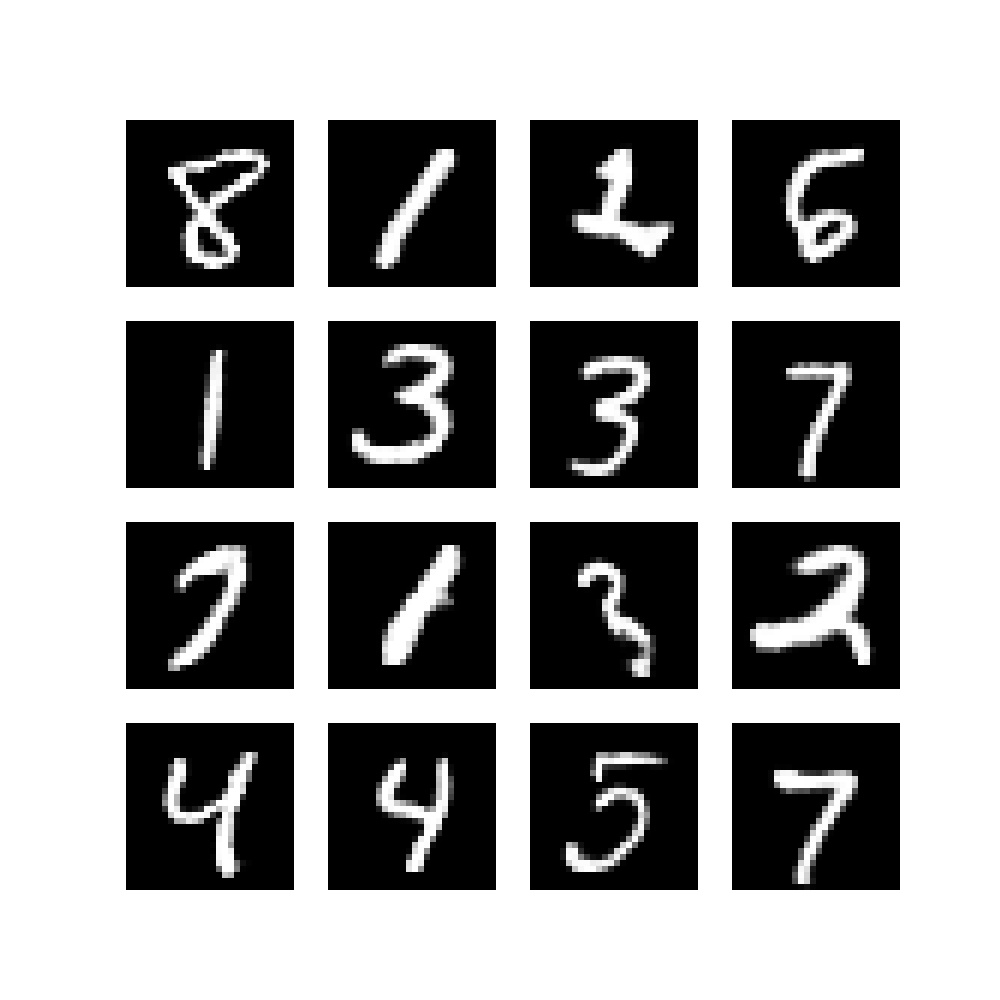•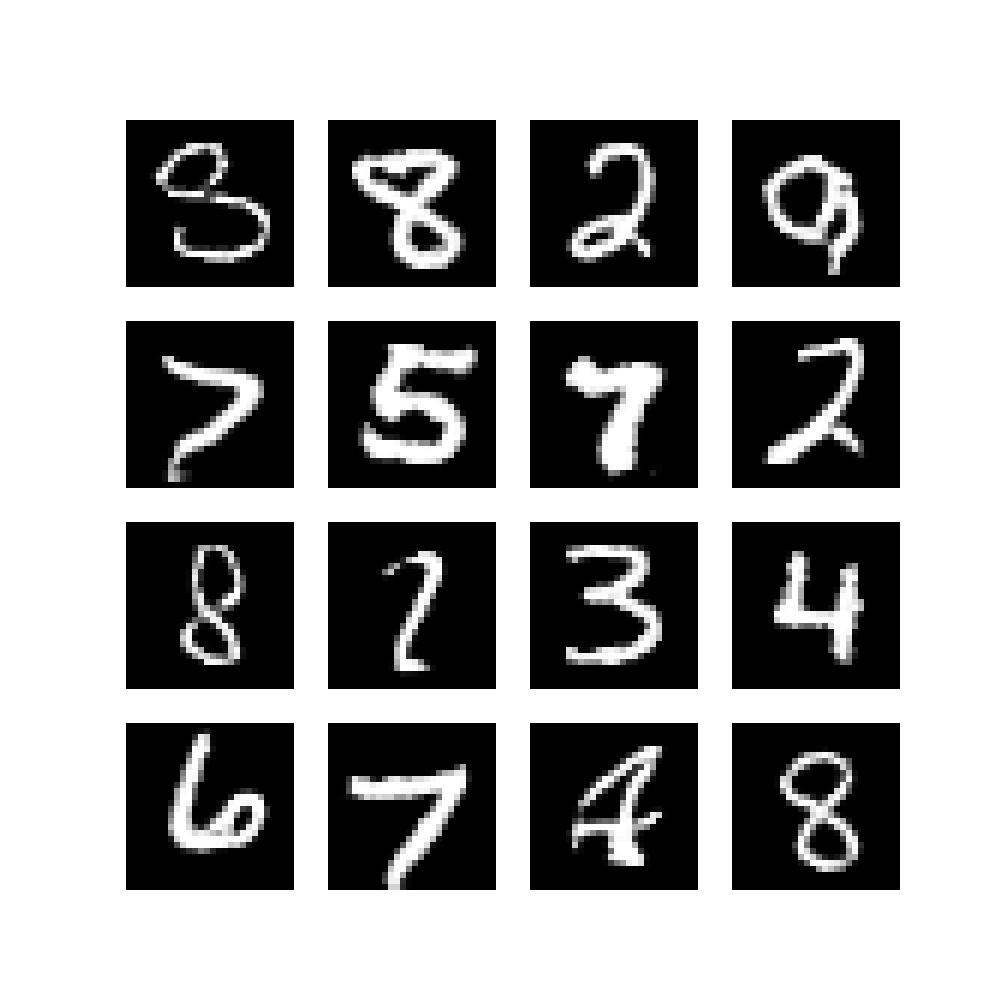•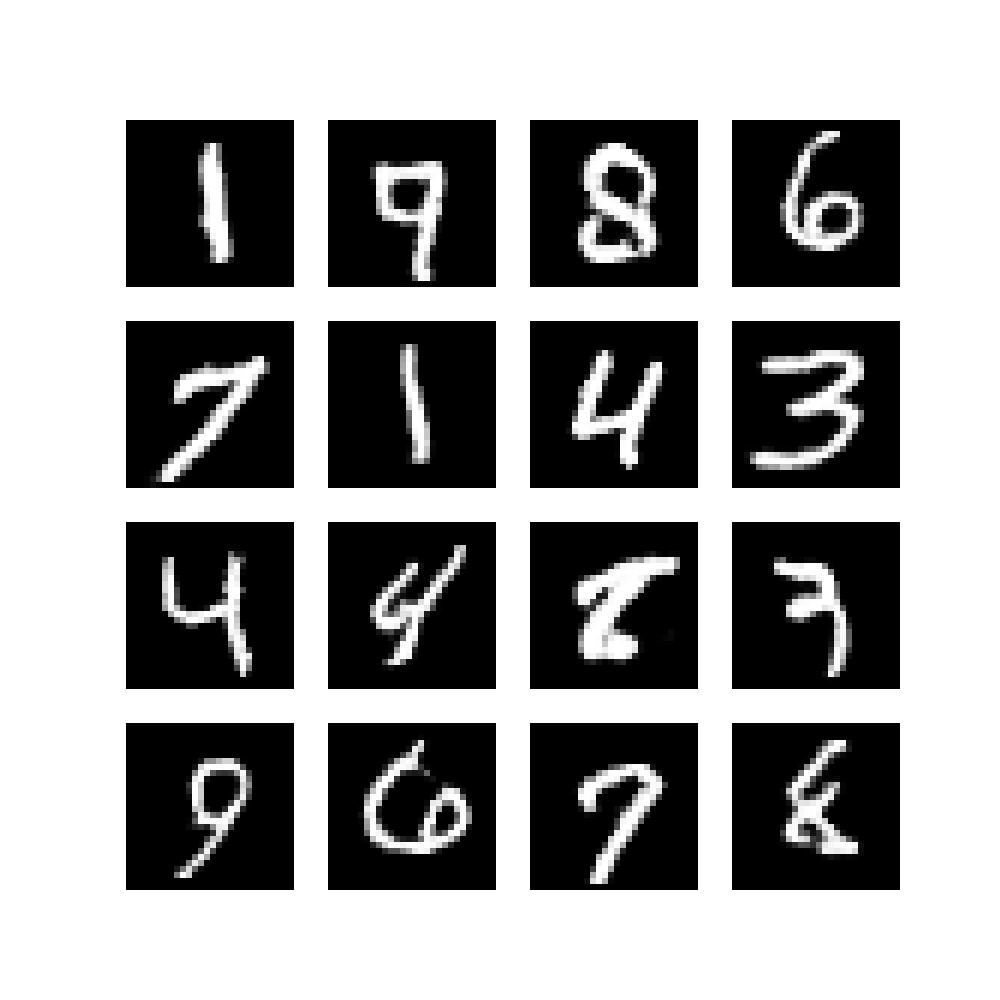•That's it, you just created a DCGAN from scratch! :)

## Summary

• Learned what a DCGAN is, to understand what is happening.
• Learned to build a DCGAN with PyTorch.
• Seen what happens when you train it on the MNIST dataset.

I hope that it was useful for your learning process! Please feel free to leave a comment in the comment section below if you have any questions or other remarks. I'll happily respond and adapt the article when necessary.

Thank you for reading MachineCurve today and happy engineering! 😎

## Sources

Radford, A., Metz, L., & Chintala, S. (2015). Unsupervised representation learning with deep convolutional generative adversarial networks. arXiv preprint arXiv:1511.06434

Verma, A. (2021, April 5). How to make your PyTorch code run faster. Medium. https://betterprogramming.pub/how-to-make-your-pytorch-code-run-faster-93079f3c1f7b

TensorFlow. (n.d.). Deep Convolutional generative adversarial networkhttps://www.tensorflow.org/tutorials/generative/dcgan